$B Multiphonic Case $B>e$X(B: HMM Using Rhythm Vectors $BLa$k(B: Rhythm Vector: a Tempo-Invariant ## Rhythm Estimation: Search Problem in HMM  a hidden state state transition output signal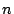-tuples of note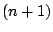-gram rhythm vectors The two probabilistic models of rhythm vector and rhythm pattern can be combined in the HMM framework as shown in Table 1 and Fig. 2. This HMM gives the probability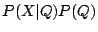, where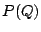is the probabilistic model of rhythm score and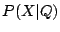is that of rhythm vectors and tempo fluctuation. Rhythm estimation is to find the time sequence of states in the state transition network,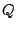, that gives the maximum a posteriori probability,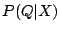, given a sequence of observed note lengths series,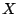. Maximizingis equivalent to maximizingaccording to Bayes theorem. The optimal sequence of states in HMMs is efficiently found through the well-known Viterbi algorithm. The sequence of intended notes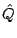is estimated in the maximum likelihood sense.$B Multiphonic Case $B>e$X(B: HMM Using Rhythm Vectors $BLa$k(B: Rhythm Vector: a Tempo-Invariant
$BJ?@.(B16$BG/(B3$B7n(B25$BF|(B